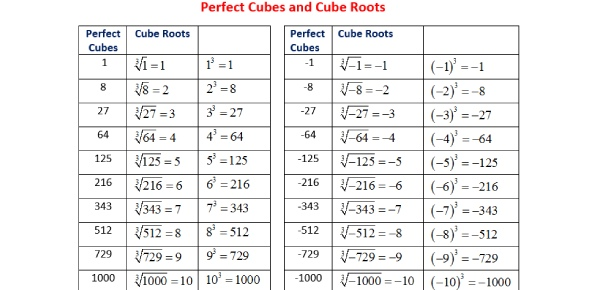# Square And Cube Roots

6 Questions | Total Attempts: 629SettingsIf you do well on this quiz you are ready for your finals.

• 1.
Wich of the following, is the cube root of 114
• A.

38

• B.

Tomatoes

• C.

5

• D.

Potatoes

• E.

Irrational between 4-5

• F.

All of the above

• 2.
The cube root of 120 is 40 because 40 x 3 = 120.
• A.

True

• B.

False

• 3.
What are the cubes of 1, 3, 5, and 8.
• A.

1

• B.

My name is Francisco

• C.

25

• D.

27

• E.

Armando .5

• F.

124

• G.

126

• H.

125

• I.

Joker

• J.

512

• 4.
What is the square root of 144
• A.

10

• B.

13

• C.

12

• 5.
What is the square root of 25
• A.

7

• B.

9

• C.

5

• 6.
What are the squres of 4-8
• A.

16

• B.

25

• C.

36

• D.

49

• E.

64

• F.

12

• G.

98

• H.

31

• I.

63

Related TopicsBack to top